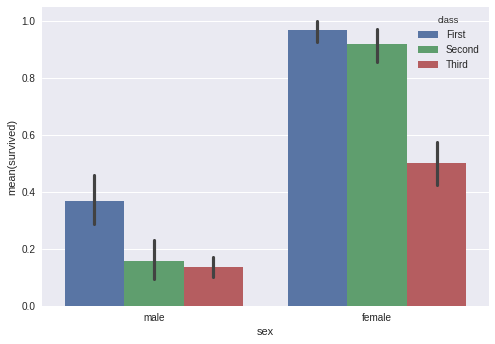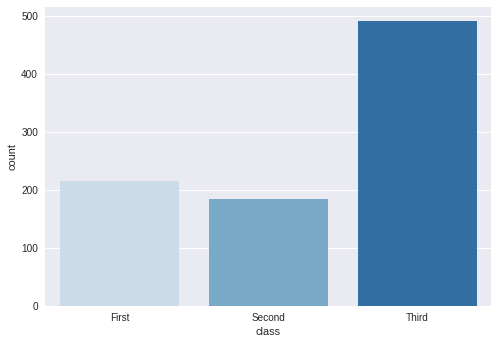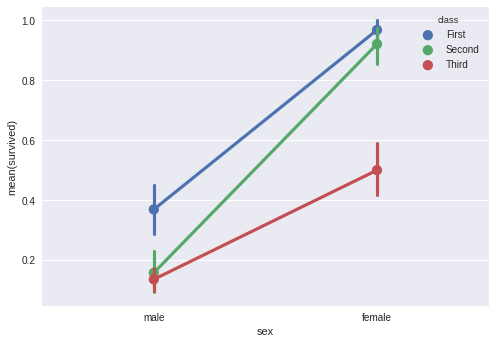# Seaborn - Working with Statistical Estimation¶

In most of the situations, we deal with estimations of the whole distribution of the data. But when it comes to central tendency estimation, we need a specific way to summarize the distribution. Mean and median are the very often used techniques to estimate the central tendency of the distribution.

In all the plots that we learned in the above section, we made the visualization of the whole distribution. Now, let us discuss the plots with which we can estimate the central tendency of the distribution.

Bar Plot The barplot() shows the relation between a categorical variable and a continuous variable. The data is represented in rectangular bars where the length of the bar represents the proportion of the data in that category.

Bar plot represents the estimate of central tendency. Let us use the ‘titanic’ dataset to learn bar plots.

In :
import pandas as pd
import seaborn as sb
from matplotlib import pyplot as plt
sb.barplot(x = "sex", y = "survived", hue = "class", data = df)
plt.show()In the above example, we can see that the average number of survivals of males and females in each class. From the plot, we can understand that the number of females survived than males. In both males and females, a number of survivals are from first class.

A special case in barplot is to show the no of observations in each category rather than computing a statistic for a second variable. For this, we use countplot().

In :
import pandas as pd
import seaborn as sb
from matplotlib import pyplot as plt
sb.countplot(x = "class", data = df, palette = "Blues");
plt.show()Plot says that, the number of passengers in the third class are higher than first and second class.

### Point Plots¶

Point Plots serve the same as bar plots but in a different style. Rather than the full bar, the value of the estimate is represented by the point at a certain height on the other axis.

In :
import pandas as pd
import seaborn as sb
from matplotlib import pyplot as pltDolly Solanki